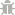Light Mode
Rate us!# GPA Calculator

Course
CreditsThe GPA calculator on SmallSEOTools is an online utility for the assistance of students in calculating GPA. Whether you want to evaluate your GPA for a certain course or an entire semester, you can get your hands on this grade point average calculator and get accurate results instantaneously. Students frequently discuss their upcoming semester's GPA with their fellows or estimate their GPA using wild estimations. Instead of frustrating yourself, you can turn to the online GPA calculator and calculate your GPA without any hassle.

### Calculate GPA without Restrictions

The grade point average calculator helps you perform gpa calculation as many times as you want without imposing any restrictions. This tool is compatible with all kinds of devices; you can use it to calculate your GPA anytime, anywhere.

## How to calculate gpa online?

The process of GPA calculation is quite easy using our GPA calculator online. Just follow the simple steps given below:

1. Enter the Course Name you have taken in a semester in the first column.

2. Enter Grades for Each Course that you expect or have obtained.

3. Enter Credits for Each Course of the semester.

4. Click the Calculate Button to get the GPA calculations in a matter of seconds.

## Basic GPA Calculation Formula

Calculating GPA on your own can be an extremely tiring process. You have to invest time and effort in order to calculate your GPA accurately.

Here is the formula you can use to calculate GPA:

Semester GPA = Total Grade Points / Total Credit Hours

To calculate your GPA with this formula, you have to calculate your grade points first. The grade points are calculated by multiplying the course of individual grades with the number of credits hours. After that, the results are added to get a value for total grade points. Once it is done, you need to divide this figure by the total number of credit hours. Its result will be your GPA for the semester.

Instead of going through the hassle involved in calculating gpa, just bookmark this grade point average calculator and use it whenever you want! If you have any suggestions for the betterment of the GPA calculator, you can feel free to contact us. We will surely work on implementing them!

## Grades and Quality Points Used by this Calculator

GPA is a common indicator used by universities all over the globe to assess and evaluate the academic achievement of students. The grades in the alphabet are converted to numeric values by this semester gpa calculator to help you count GPA. The table given below shows how our gpa counter shows your grades to numeric values:

 Grades Numeric Equivalents A+ 4.3 A 4 A- 3.7 B+ 3.3 B 3 B- 2.7 C+ 2.3 C 2 C- 1.7 D+ 1.3 D 1 D- 0.7 F 0

## FAQs

### How to calculate gpa of semester?

You can calculate letter grade to gpa of any semester with the help of our semester GPA calculator available on SmallSEOTools. By simply submitting your course grades and credit hours, this tool allows you to assess your overall GPA instantly!

### What is a Good GPA for University?

A good GPA for a university varies from institute to institute. A 3.0 or above GPA is generally considered good for universities, as it stands you at an average “B” grade and gives you eligibility to apply to a wide range of schools.

### Is a 3.7 GPA an Average?

As per most institutes, a 3.7 GPA is considered an “A-” in grades for the academic performance of the students.

### Can I Get into Harvard with a 3.7 GPA?

Normally, 3.7 is considered is a good GPA by many universities. Harvard doesn’t have a requirement for a minimum GPA, but due to its high competitiveness, the average GPA to get into Harvard is 3.9.

### Is 1.83 a Good GPA?

No! a 1.83 GPA isn’t a good GPA at all, as it is below average. 3.0 is considered an average GPA, so anything below it isn’t good. That person must put a lot of efforts to improve their GPA.

### What Will Be My GPA After This Semester?

Your GPA after this semester depends upon the grades you have scored in the selected courses and the number of credit hours in them. Take advantage of semester GPA calculator to know your estimated GPA.

### How to Calculate your overall GPA?

Your overall GPA is calculated by dividing your cumulative course hours by your cumulative points. Use our overall GPA calculator and input the hours and points earned in prior courses. The hours, points, and GPA from your most recent semester will be immediately added.

### AS SEEN ON:

Plagiarism Checker